The radius of a Triangle's Incircle or of a Polyhedron's Insphere, denoted. For a Triangle,(1)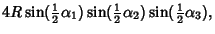(2)

whereis the Area of the Triangle,,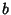, and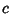are the side lengths,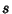is the Semiperimeter, andis the Circumradius (Johnson 1929, p. 189).

Equation (1) can be derived easily using Trilinear Coordinates. Since the Incenter is equally spaced from all three sides, its trilinear coordinates are 1:1:1, and its exact trilinear coordinates are. The ratioof the exact trilinears to the homogeneous coordinates is given by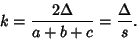(3)

But sincein this case,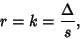(4)

Q. E. D.

Other equations involving the inradius include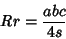(5)(6)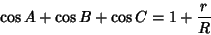(7)(8)(9)

where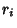are the Exradii (Johnson 1929, pp. 189-191).

As shown in Right Triangle, the inradius of a Right Triangle of integral side lengths,, andis also integral, and is given by(10)

whereis the Hypotenuse. Letbe the distance between inradiusand Circumradius,. Then(11)(12)

(Mackay 1886-87). These and many other identities are given in Johnson (1929, pp. 186-190).

Expressing the Midradiusand Circumradiusin terms of the midradius gives(13)(14)

for an Archimedean Solid.

References

Johnson, R. A. Modern Geometry: An Elementary Treatise on the Geometry of the Triangle and the Circle. Boston, MA: Houghton Mifflin, 1929.

Mackay, J. S. Historical Notes on a Geometrical Theorem and its Developments [18th Century].'' Proc. Edinburgh Math. Soc. 5, 62-78, 1886-1887.

Mackay, J. S. Formulas Connected with the Radii of the Incircle and Excircles of a Triangle.'' Proc. Edinburgh Math. Soc. 12, 86-105.

Mackay, J. S. Formulas Connected with the Radii of the Incircle and Excircles of a Triangle.'' Proc. Edinburgh Math. Soc. 13, 103-104.